• 图形旋转平移缩放， 几何变换是指把一个几何图形变换成另一个几何图形的方法，若仅改变图形的位置，而不改变图形的形状大小，这种变换称为合同变换，平移旋转是常见的合同变换．旋转平移缩放
• 图形学变换一、概述二、平移新的改变功能快捷键合理的创建标题，有助于目录的生成如何改变文本的样式插入链接与图片如何插入一段漂亮的代码片生成一个适合你的列表创建一个表格设定内容居中、居左、居右SmartyPants...

一、概述

在齐次坐标中，所有的仿射变换都可以使用如下形式的 4 x 4 矩阵来表示p ( x , y , z ) p (x, y, z) 表示方式为向量 a ⃗ ( x , y , z ) \vec a (x, y, z) 表示方式为关于齐次坐标的理解参考博文：齐次坐标

二、平移

平移（translation）变换把点沿着给定方向移动固定距离， p ′ = p + d p' = p + d 如下图所示。比如：将点 p ( 1 , 1 , 1 ) p (1, 1, 1) ，往 X X 轴正方向移动 1单位，往 Y Y 轴正方向移动 2 单位， 往 Z Z 轴正方向移动 3 单位，最终坐标是 p ′ ( 2 , 3 , 4 ) p'(2, 3, 4)
数学上的运算为 p ( 1 , 1 , 1 ) + d ( 1 , 2 , 3 ) = p ′ ( 2 , 3 , 4 ) p (1, 1, 1) + d (1, 2, 3) = p'(2, 3, 4)
在图形学中， p + d p + d 可以表示为 p p 乘于 d d 对应的平移矩阵 T T所以 p ′ p' 的平移运算可表示为二、旋转

旋转（rotation）变换指点绕着特定的一个点旋转固定角度。下面我们使用 2D 坐标讲解点 p ( x , y ) p(x, y) ，绕特定的点 O ( 0 , 0 ) O(0, 0) 原点旋转 θ θ 度，变换后的坐标表示为 p ′ ( x ′ , y ′ ) p'(x', y')p p p ′ p' 转换为极坐标表示则有利用两角和的正弦和余弦公式可得转换为矩阵像形式在 3D 中绕 Z Z 轴旋转的所有点 z z 值不变，等价于上面 2D 绕原点的旋转，所以 3D 坐标的旋转可以表示为在图形学中 绕 Z Z 轴旋转的变换矩阵为最终 p ′ p' 的旋转可表示为同理可得绕 X X 轴旋转的变换矩阵为 R x R_{x} ，绕 Y Y 轴旋转的变换矩阵为 R y R_{y} 。注：这里使用右手坐标系三、缩放

缩放（scaling）变换指沿着指定不动点（变换后该点位置保持不变，这里使用原点），对象沿着指定方向（坐标轴）伸长或缩短。缩放后的点 p ′ ( x ′ , y ′ , z ′ ) p'(x', y', z') 的坐标可表示为使用齐次坐标表示为欢迎关注个人公众号，实时推送最新博文！展开全文矩阵
• 图形的平移旋转 知识点一平移平移作图 1平移的概念及性质 (1) 平移的概念在平面内将一个图形沿某个方向移动一定的距离这样的图形运动 称为平移平移不改变图形的形状大小 2平移的特点 图形上的每一个点沿同一...
• 平移的概念在平面内将一个图形沿某个方向移动一定的距离这样的图形运动 称为平移平移不改变图形的形状大小 2平移的特点 图形上的每一个点沿同一个方向移动相同的距离 平移不改变图形的形状大小方向只改变图形的...
• } void CGeotranView::Ontranslation() { // 平移 tx=50,ty=60 CDC *pDC=GetDC(); int tx=50,ty=60; pDC->MoveTo(100+tx,100+ty); pDC->LineTo(200+tx,50+ty); pDC->LineTo(200+tx,150+ty); pDC->LineTo(100+tx,100...

以下均为MFC工程代码

代码一：简单实现

void CGeotranView::Onyuantu()

{

// 原图，画三角形

CDC *pDC=GetDC();

pDC->MoveTo(100,100);

pDC->LineTo(200,50);

pDC->LineTo(200,150);

pDC->LineTo(100,100);

ReleaseDC(pDC);

}

void CGeotranView::Ontranslation()

{

// 平移 tx=50,ty=60

CDC *pDC=GetDC();

int tx=50,ty=60;

pDC->MoveTo(100+tx,100+ty);

pDC->LineTo(200+tx,50+ty);

pDC->LineTo(200+tx,150+ty);

pDC->LineTo(100+tx,100+ty);

ReleaseDC(pDC);

}

void CGeotranView::Onrotation()

{

// 旋转, 角度sita=30度

CDC *pDC=GetDC();

double sita=30;

double hudu=sita*3.14159/180;

pDC->MoveTo(int(100*cos(hudu)-100*sin(hudu)),int(100*sin(hudu)+100*cos(hudu)));

pDC->LineTo(int(200*cos(hudu)-50*sin(hudu)),int(200*sin(hudu)+50*cos(hudu)));

pDC->LineTo(int(200*cos(hudu)-150*sin(hudu)),int(200*sin(hudu)+150*cos(hudu)));

pDC->LineTo(int(100*cos(hudu)-100*sin(hudu)),int(100*sin(hudu)+100*cos(hudu)));

ReleaseDC(pDC);

}

void CGeotranView::Onscaling()

{

// 缩放 sx=2,sy=3

int sx=2,sy=3;

CDC *pDC=GetDC();

pDC->MoveTo(100*sx,100*sy);

pDC->LineTo(200*sx,50*sy);

pDC->LineTo(200*sx,150*sy);

pDC->LineTo(100*sx,100*sy);

ReleaseDC(pDC);

}

代码二：新的点的坐标计算通过函数实现

POINT translationPoint(POINT point,int tx,int ty)

{

POINT newpoint;

newpoint.x=point.x+tx;

newpoint.y=point.y+ty;

return newpoint;

}

POINT rotationPoint(POINT point,double sita)

{

POINT newpoint;

double hudu=sita*3.14159/180;

newpoint.x=(int)(point.x*cos(hudu)-point.y*sin(hudu));

newpoint.y=(int)(point.x*sin(hudu)+point.y*cos(hudu));

return newpoint;

}

POINT scalingPoint(POINT point,int sx,int sy)

{

POINT newpoint;

newpoint.x=point.x*sx;

newpoint.y=point.y*sy;

return newpoint;

}

void CGeotranView::Onyuantu2()

{

POINT point1,point2,point3;

point1.x=200;point1.y=200;

point2.x=300;point3.y=150;

point3.x=300;point2.y=250;

// 原图，画三角形

CDC *pDC=GetDC();

pDC->MoveTo(point1);

pDC->LineTo(point2);

pDC->LineTo(point3);

pDC->LineTo(point1);

ReleaseDC(pDC);

}

void CGeotranView::Ontranslation2()

{

POINT point1,point2,point3;

point1.x=200;point1.y=200;

point2.x=300;point3.y=150;

point3.x=300;point2.y=250;

// 平移 tx=50,ty=60

CDC *pDC=GetDC();

int tx=50,ty=60;

pDC->MoveTo(translationPoint(point1,tx,ty));

pDC->LineTo(translationPoint(point2,tx,ty));

pDC->LineTo(translationPoint(point3,tx,ty));

pDC->LineTo(translationPoint(point1,tx,ty));

ReleaseDC(pDC);

}

void CGeotranView::Onrotation2()

{

POINT point1,point2,point3;

point1.x=200;point1.y=200;

point2.x=300;point3.y=150;

point3.x=300;point2.y=250;

// 旋转, 角度sita=30度

CDC *pDC=GetDC();

double sita=30;

pDC->MoveTo(rotationPoint(point1,sita));

pDC->LineTo(rotationPoint(point2,sita));

pDC->LineTo(rotationPoint(point3,sita));

pDC->LineTo(rotationPoint(point1,sita));

ReleaseDC(pDC);

}

void CGeotranView::Onscaling2()

{

POINT point1,point2,point3;

point1.x=200;point1.y=200;

point2.x=300;point3.y=150;

point3.x=300;point2.y=250;

// 缩放 sx=2,sy=2

int sx=2,sy=2;

CDC *pDC=GetDC();

pDC->MoveTo(scalingPoint(point1,sx,sy));

pDC->LineTo(scalingPoint(point2,sx,sy));

pDC->LineTo(scalingPoint(point3,sx,sy));

pDC->LineTo(scalingPoint(point1,sx,sy));

ReleaseDC(pDC);

}

展开全文• 1、平移变换三维图形平移变换可以描述为： %% 圆的平移，x加1，y加1，z+1clc;clear all;figure(1);axis equal;sphere(50);%球由50*50个面组成[x y z]=sphere();hold on;dx = 1;dy = 1;dz = 1;%坐标加一T = [1 0 0 ...

1、平移变换

三维图形的平移变换可以描述为：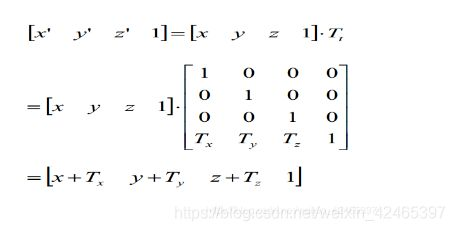%% 圆的平移，x加1，y加1，z+1

clc;clear all;

figure(1);

axis equal;

sphere(50);%球由50*50个面组成

[x y z]=sphere();

hold on;

dx = 1;dy = 1;dz = 1;

%坐标加一

T = [1 0 0 0;0 1 0 0;0 0 1 0;dx dy dz 1];

Ex = x.*T(1,1)+y.*T(2,1)+z.*T(3,1)+1.*T(4,1);

Ey = x.*T(1,2)+y.*T(2,2)+z.*T(3,2)+1.*T(4,2);

Ez = x.*T(1,3)+y.*T(2,3)+z.*T(3,3)+1.*T(4,3);

% surf(E(:,1:21),E(:,22:42),E(:,43:63));

%surf(x+dx,y+dy,z+dz);

surf(Ex,Ey,Ez);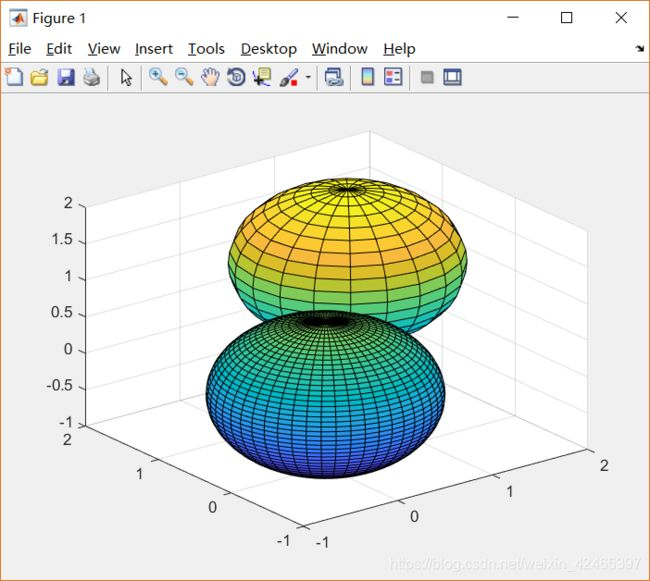2.旋转变换

三维图形的旋转变换可描述为：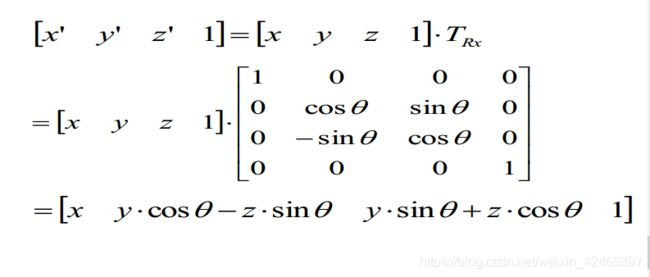%% 绘制一个柱体，然后绕x轴旋转60°

clear all;clc;

[x,y,z]=cylinder(3); %调用cylinder函数‘()’内半径为3. 运行此命令后系统自动为x,y,z填充了数据

z(2,:)=2; %设置矩阵Z的第二行数值全为2，即设置圆柱体高度

figure;

surf(x,y,z,'FaceColor',[1,0,0]); %使用surf功能将x,y,z的数据导入。FaceColor即设置圆柱体颜色，红色色号为[1,0,0]

daspect([1 1 1]) %设置x,y,z坐标间距等长。

view(30,30) %设置图像显示角度

hold on

theta = linspace(0,2*pi,40) %将一个圆周分为40份

X=3*cos(theta); %将cos值赋予x

Y=3*sin(theta); %将sin赋予Y

Z=ones(size(X))+1; %设置Z与X长度想等

fill3(X,Y,Z,[1,0,0]); %使用Fill3功能函数，填充XYZ所围成的区域，这个是顶盖

fill3(X,Y,Z-2,[1,0,0]); %底盖

hold on

T = [1 0 0 0;0 cosd(60) sind(60) 0;0 -sind(60) cosd(60) 0;0 0 0 1];

Ex = x.*T(1,1)+y.*T(2,1)+z.*T(3,1)+1.*T(4,1);%这三句是旋转的柱面的侧面

Ey = x.*T(1,2)+y.*T(2,2)+z.*T(3,2)+1.*T(4,2);

Ez = x.*T(1,3)+y.*T(2,3)+z.*T(3,3)+1.*T(4,3);

%下面是旋转圆面，只旋转的上面的圆面，下面的没有旋转

Ex1 =X.*T(1,1)+Y.*T(2,1)+Z.*T(3,1)+1.*T(4,1);

Ey1 = X.*T(1,2)+Y.*T(2,2)+Z.*T(3,2)+1.*T(4,2);

Ez1 = X.*T(1,3)+Y.*T(2,3)+Z.*T(3,3)+1.*T(4,3);

surf(Ex,Ey,Ez);

fill3(Ex1,Ey1,Ez1,[1,0,0]);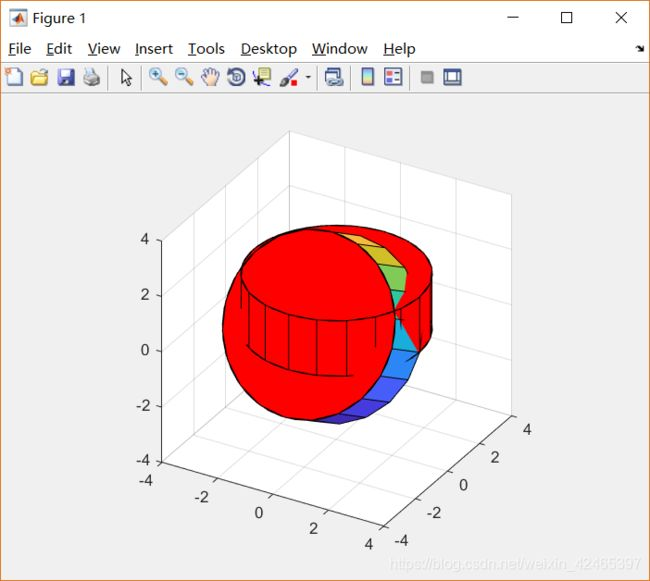3.错切变换

错切变换的常用变换矩阵为：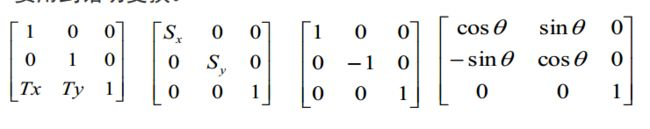%% 3.用sphere生成多面体，然后对其进行错切变换，不断的改变错切系数和多面体的数量

clear all;clc;

T=[1 0 0;

1 1 0;

0 0 1];

for num = 1:10 %多边形的数量

for ti = 1:0.05:1.1 %错切系数

T(2,1) = T(2,1)*ti; %改变错切系数

[x,y,z] = sphere(num);

fprintf("错切系数：%f\n",T(2,1));

surf(x,y,z);

fprintf("显示第%d边形\n",num);

pause(0.5);

for i=1:num+1

for j=1:num+1

a=x(i,j);

b=y(i,j);

c=z(i,j);

m=[a b c]*T';

x1(i,j)=m(1);

y1(i,j)=m(2);

z1(i,j)=m(3);

end

end

fprintf("错切第%d边形\n",num);

surf(x1,y1,z1)

pause(0.5);

end

T(2,1) = 1;

end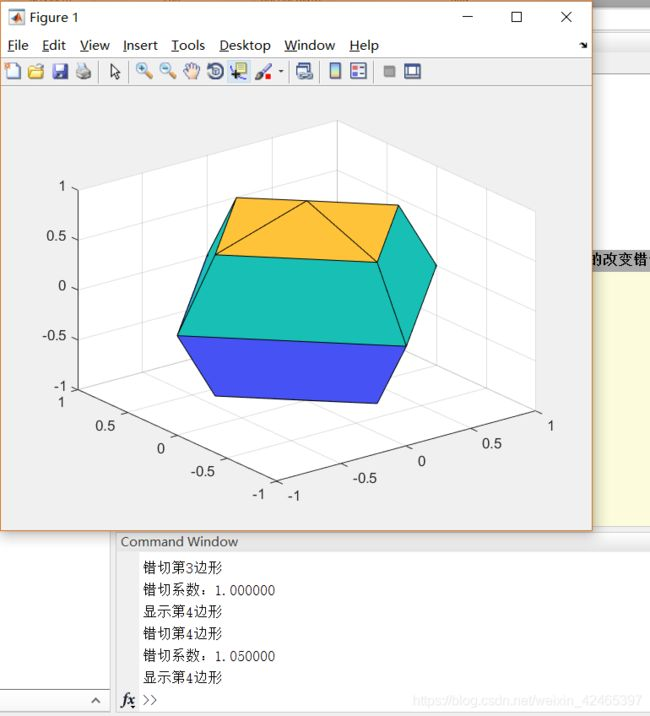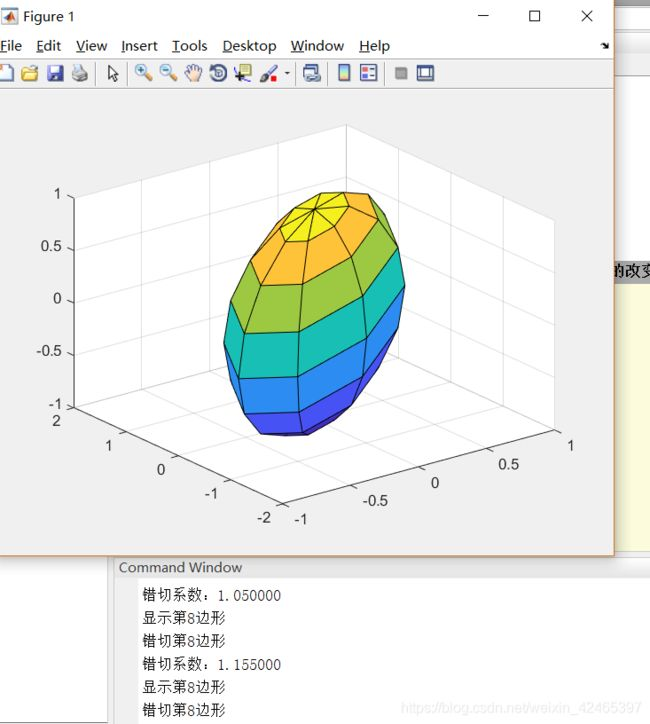展开全文• 图像几何变换又叫做图像基本变换，主要包括图像平移、图像缩放图像旋转几个部分，当然还有图像镜像等简单的内容。图像基本变换是图像处理的基本内容，是学习以后复杂的仿射变换、透视变换以及更高级的MLS网格变形...

本篇作为新年到来前的最后一篇，提前祝大家新年快乐！

图像几何变换又叫做图像基本变换，主要包括图像平移、图像缩放和图像旋转几个部分，当然还有图像镜像等简单的内容。图像基本变换是图像处理的基本内容，是学习以后复杂的仿射变换、透视变换以及更高级的MLS网格变形等内容的基础，意义重大。本篇将从平移、缩放和旋转三个方面来讲解如何单纯使用C语言来轻松实现这三个算法。

图像平移变换

[定义与算法]

图像平移变换可以表示为水平方向和垂直方向的位移，如果把图像坐标系的原点(0,0)点平移到(x0,y0)，则图像内任意一点(x,y)平移后坐标(x’,y’)用公式表示如下：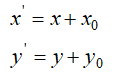我们对测试图进行水平和垂直正方向平移100像素，效果图如图Fig.1所示。

注意，黑色区域是我们默认填充的颜色，平移变换会出现图像跑到原图画布外面的情况，此时，原来的区域可以填充任意颜色，图像平移变换就这么简单。

展开全文图像旋转 图像平移 图像缩放 C源代码
• 二维图形学的变换-平移、旋转、缩放 OpenGL

万次阅读 多人点赞 2016-12-14 13:34:28
这里实现的是多点画多边形，然后把这个多边形进行二维的变换。 首先，多点画多边形，为了方便起见，我直接调用...假如我要平移a到b的位置： 那么操作就是：把这个多边形的n个顶点从一个位置移动到另外一个位置，然后变换 多边形 缩放
• 计算机图形学与计算机视觉、数字图像处理的区别 计算机图形学，输入的是结构特征数据，输出的是图像信号； 计算机视觉，输入的是图像信号，输出的是结构特征数据； 数字图像处理，输入的是图像信号，输出的也是图像...
• 3.第二条中操作包括：旋转平移、缩放 该文章：首先介绍一个图形时候的情况。多个图形不断更新。 环境 我是用xcode写的。但是不用担心，你需要修改的或许只是include头文件的两个字母。 基础知识： 你会在...计算机图形学 鼠标键盘 交互
• 本文主要讲述基于VC++6.0 MFC图像处理的应用知识，主要结合自己大三所学课程《数字图像处理》及课件进行讲解，主要通过MFC单文档视图实现显示BMP图片空间几何变换，包括图像平移图形旋转、图像反转倒置镜像图像...MFC 数字图像处理 图像平移
• 旋转平移、伸缩与剪切 绕任意轴旋转 注：Coordinate systems and frames，本文译为坐标系与广义坐标系。 一、坐标系与广义坐标系 1.1 坐标系旋转变换 向量 v∈R3v \in R^3v∈R3 可以用一组基向量v1,v2,v3v_1, v_...
• 二维图像的基本几何变换主要包括镜像、平移、缩放、旋转、错切(偏移)等操作，上述变换又称为仿射变换，在冈萨雷斯的数字图像处理第三版的第二章就做了相关介绍，数字图像处理第三版下载地址。    &...
• 计算机图形学作业（ 三）：使用openGL画一个立方体，并实现平移旋转和放缩变换题目引入GLM库画立方体模型、观察投影 题目 引入GLM库 利用openGL进行3D绘图需要用到大量的数学矩阵运算，而OpenGL没有自带...openGL
• 介绍三种主要的几何变换：平移、缩放、旋转平移变换 将三维空间中的一个点 [x,y,z,1][x, y, z, 1][x,y,z,1] 移动到另外一个点 [x′,y′,z′,1][x', y', z', 1][x′,y′,z′,1] ，三个坐标轴的移动分量分别为 dx=...
• 二维几何变换（平移旋转、缩放）本章涉及数学变换比较多，代码是次要的，数学理论可自己推导一下。【二维平移】通过将二维量加到一个点的坐标上来生成一个新的坐标位置，可以实现一次平移。将平移距离加到原始坐标...计算机图形 OpenGL
• 但是，模型平移一个距离后，再次旋转时，发现旋转中心在模型的中心了，导致旋转非常别扭。 问题现象对应的绘图代码如下： glMatrixMode(GL_MODELVIEW); glLoadIdentity();// Reset the coordinate system ...
• 5.1 向量基础知识我们所使用的所有点向量是基于某一坐标系定义的，比如左手坐标系或者右手坐标系。从几何的角度来看，向量是具有长度方向的实体，但是没有位置，而点是只有位置，没有长度方向。在几何中，把...计算机图形学 二维图形的几何变换
• 1.有关变换 1.1平移变换 rigid-body transformation产生形变的移动物体的刚体变换，为P点沿着直线从一个坐标位置到另一个坐标位置的过程，例图： 推导过程： 结果的平移变换矩阵：windows 矩阵
• Matlab 图像平移、旋转、缩放、镜像

千次阅读 多人点赞 2019-09-27 15:46:21
对图像进行缩放、平移旋转可以转化为矩阵的运算。关于变换矩阵的构造，请参考：《[gym 101047C Robotics Competition] 矩阵快速幂求解点旋转平移N次之后的位置》参考原图： 1. 图像平移 init = imr...
• 仿射变换原理 仿射变换（Affine Transformation或 Affine Map...一个任意的仿射变换能表示为乘以一个矩阵(线性变换)接着再加上一个向量(平移)的形式。可以表示为线性变换和平移变换的叠加 我们能够用仿射变换来表示计算机视觉 opencv
• MFC+OPENGL配置+显示三维图形实现 旋转平移缩放+光照效果[对话框篇] 一、开发环境说明 操作系统：windows 开发软件：VS2017 编程语言：基于MFC对话框下的opengl 最终效果图： 二、配置操作 配置opengl...opengl mfc
• 本专栏所有文章示例代码均可在我的git码云上获取，读者可自行下载：https://gitee.com/babyogl/learnWebGL,本文示例代码在chapter-02的color-triangle-01,引用文件在libs文件夹下；在上一节中，笔者介绍了如何用...
• 交互式 QGraphicsView（平移/缩放/旋转）

万次阅读 多人点赞 2016-12-09 19:09:57
Graphics View提供了一个平台，用于大量自定义 2D 图元的管理与交互，框架包括一个事件传播架构，支持场景 Scene 中的... Google 地图一样，在管理大量 Item 的时候，通常需要 View 具有交互（平移/缩放/旋转）功能。Qt QGraphics Qt图形视图 Graphics缩放
• 2.旋转Cow到背面 3.想看清楚Cow面部的细节，改变它大小等等 可能你会说，这还简单，通过操作相机就好了。操作相机，使得相机的空间位置发生了变化，但对三维物体的空间位置并没有改变，要想改变模型的空间位置，...VTK Transform 空间变换
• 欢迎大家来到“Python从零到壹”，在这里我将分享约200篇Python系列文章，带大家一起去学习...这篇文章将详细讲解图像几何变换，包括图像平移、图像缩放图像旋转。希望文章对您有所帮助，如果有不足之处，还请海涵。python 图像处理 OpenCV 几何变换
• 本文主要讲述基于VC++6.0 MFC图像处理的应用知识，主要结合自己大三所学课程《数字图像处理》及课件进行讲解，主要通过MFC单文档视图实现显示BMP图片空间几何变换，包括图像平移图形旋转、图像反转倒置镜像图像...MFC 图像处理 几何变换 图像平移旋转 图像镜像
• 几何变换不改变图像的像素值， 只是在图像平面上进行像素的重新安排。适当的几何变换可以最大程度地消除由于成像角度、透视关系乃至镜头自身原因所造成的几何失真所产生的负面影响。几何变换常常作为图像处...缩放 仿射变换 透视变换
• 平移不变性:不管出现在图像中的哪个位置，神经网络的底层应该对相同的图像区域做出类似的响应。 这个原理即为“平移不变性”。 2. 局部性:神经网络的底层应该只探索输入图像中的局部区域，而考虑图像远处区域的...图像处理...